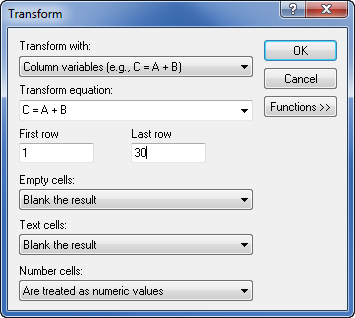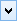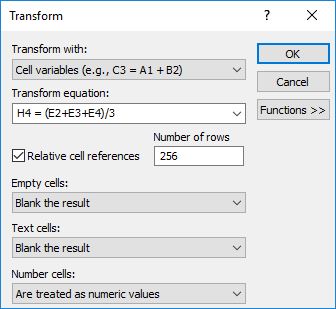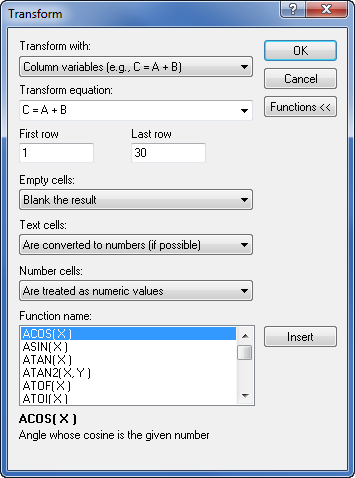# Transform - Worksheet

Click the Data Tools | Data | Transform command to open the Transform dialog, where you can apply mathematical transformations to columns, rows, or cells. Valid math operators include addition (+), subtraction (-), multiplication (*), and division (/) as well as a large library of built-in mathematical functions. Parentheses should be used to override precedence or for clarification.Use the Transform dialog to apply math functions to data.

The dialog options update to reflect the option selected for

Transform with field.

## Transform With

Select the type of transform from the Transform with list.

• Column variables (e.g., C = A + B) applies the transform equation to the specified rows in the Transform equation column.

• Row variables (i.e., _3 = _1 + _2) applies the transform equation to the specified columns in the Transform equation row.

• Cell variables (i.e., C3 = A1 + B2) applies the transform equation to the cell specified in the Transform equation. Multiple cells can be transformed by selecting the Relative cell references option.

## Transform Equation

Type the formula into the Transform equation box. Formulas consist of a destination column, row, or cell on the left side of the equation and a mathematical manipulation on the right side of the equation. Use the column label letters, row numbers, or cell locations on both sides of the equation. Click the down arrow to use previously entered equations. For columns, a sample equation may be C = A + B. For rows, a sample equation is _4=_1+_2. For cells, a sample equation would look like C2=A1+B1-C1.

If the transform method is by column, the range functions (sum, avg, std, rowmin and rowmax) take column indices only, i.e., sum(A...C). If transform method is by variable rows, the range functions take row indices only, i.e., sum(_1..._3). If transform method is by variable cells, the range functions are not supported.

The last ten functions are stored in the Transform equation field. After ten functions are included in the list, the oldest function is replaced when a new function is added. The Transform equations are stored between sessions. To use a stored function, click thein the Transform equation box and select a function from the list. Note that the First row and Last row or First col and Last col values are not saved with the stored Transform equations.

## First and Last Columns and Rows

When calculating transformations on columns, enter the First row and the Last row to limit the calculation to the specified rows. When calculating transformations on rows, enter the First col and Last col to limit the calculation to the specified columns. When calculating transformations on cells, the First row, Last row, First col, and Last col options are not available.

By default, these are set to the first row and last row (or first column and last column) with text or numbers entered into a cell for the entire worksheet.

## Relative Cell References

Select the Relative cell references option to apply the Transform equation to multiple cells. The Relative cell references option is only available when Transform with is set to Cell variables (e.g., C3 = A1 + B2). The Transform equation is only applied relatively by incrementing the row numbers. The columns do not change when Relative cell references is selected.The Relative cell references option applies the Transform equation to multiple rows.

Specify the number of rows to which the Transform equation is applied in the Number of rows field. Note this value is not the row number. For example in the image above, the Transform equation starts at cell H4 with the equation H4 = (E2+E3+E4)/3 and transforms a total of 256 rows, ending at cell H259. By default the Number of rows value is the total number of rows in the worksheet.

## Empty Cells

The Empty cells option controls how empty cells are treated in the calculations of formulas. Available options are Blank the result, Are treated as the number zero (0), and Are treated as empty text (""). The default option is Blank the result, which results in the formula not being calculated for any row that contains a blank cell in any of transform equation rows or columns.

• Setting the Empty cells option to Blank the result results in a blank cell for the transform when the cells on the right side of the equation are empty.

• Setting the Empty cells option to Are treated as the number zero (0) results in the transform creating a number when all of the cells on the right side of the equation are empty or numeric. When the right side of the equation combines text and blank cells, the equation is blank.

• Setting the Empty cells option to Are treated as empty text ("") results in the transform creating a text string when all of the cells on the right side of the equation are empty or text. When the right side of the equation combines numeric and blank cells, the equation is blank.

## Text Cells

The Text cells option controls how text cells are treated in the calculations of formulas. Available options are Blank the result, Are treated as text, Are converted to numbers (if possible), and Are treated as the number zero (0). The default option is Blank the result, which results in the formula not being calculated for any row that contains a text cell in any of transform equation rows or columns.

• Setting the Text cells option to Blank the result results in a blank cell for the transform when any of the cells on the right side of the equation contain text strings (including numbers formatted as text).

• Setting the Text cells option to Are treated as text results in the transform creating a text string when all of the cells on the right side of the equation are text (or treated as text). If a mix of text cells and numbers or empty cells (that are not treated as text) are in the cells on the right side of the equation, the transform results in a blank cell. This option allows text strings to be concatenated.

• Setting the Text cells option to Are converted to numbers (if possible) results in the transform creating a number when all of the cells on the right side of the equation are numeric or treated as numbers. Any cells with numbers formatted as text are treated as the number. For example, the text string '05 would be treated as the number 5 if this option is selected.

• Setting the Text cells option to Are treated as the number zero (0) results in the transform creating a number when all of the cells on the right side of the equation are numeric or treated as numbers. Any cells with text are replaced with the value zero for the transform. For example, if you are using the equation C=A+B and A has Colorado and B has 45, the value in cell C will be 45.

## Number Cells

The Number cells option controls how numeric cells are treated in the calculations of formulas. Available options are Blank the result, Are treated as numeric values, Are converted to text, and Are treated as empty text (""). The default option is Are treated as numeric values, which results in the formula being calculated for any row that contains numbers in any of transform equation rows or columns.

• Setting the Number cells option to Blank the result results in a blank cell for the transform when any of the cells on the right side of the equation contain numbers. This option is useful when you only want to combine text cells or blank cells.

• Setting the Number cells option to Are treated as numeric values results in the transform creating a number when all of the cells on the right side of the equation are number (or treated as numbers). If a mix of text cells and numbers or empty cells (that are not treated as numbers) are in the cells on the right side of the equation, the transform results in a blank cell.

• Setting the Number cells option to Are converted to text results in the transform creating a text string when all of the cells on the right side of the equation are text or treated as text. Any cells with numbers are treated as the text string of the number. For example, number 5 is in the cell, so the text string would appear as '5 if this option is selected.

• Setting the Number cells option to Are treated as empty text ("") results in the transform creating a text string when all of the cells on the right side of the equation are text or treated as text. Any cells with numbers are replaced with "" for the transform. For example, if you are using the equation C=A+B and A has Colorado and B has 45, the value in cell C will be Colorado.

## Combining Text, Numbers, and Empty Cells

Many possible combinations of the Empty cells, Text cells, and Number cells exist to allow combining these different types of cells in a Transform equation. If the transform result is not what you expect, check the settings for these options and adjust if necessary.

## Functions

Click the Functions >> button to open a list of predefined mathematical functions. Click the Functions << button to hide the list of predefined mathematical functions.

To use a function, place the cursor in the location to add a function, select a function from the list, click the Insert button, and then replace the X in the function with a column letter (A), row number (_1), or cell location (A1). Also, be sure to use proper mathematical operators (+_*/) between the function and the rest of the equation. The definition of the function is listed below the Function name list when a function is selected.

Row or column range functions, for example SUM(A..Z), cannot be used with a cell variable Transform equation.

## Insert

When the Functions are expanded, the Insert button is visible. Select a function and click the Insert button to add a function to the equation. Change the variable (i.e. X) in the listed functions to a column letter, row number (_1), or cell location in the transformation equation.This example used the Functions button to choose a
predefined function from the Function name list. The Insert
button was used to add the selected function to the
Transform equation box. The values were changed to
fit the desired column variables.

## Errors

Any calculations that result in error values are listed in the Transform Errors dialog. The most common error is "floating point divide by zero."

## Examples

An example of a column formula is C = A + B. Columns A and B are added and inserted into column C with this equation. The formula adds the contents of A and B in each row and places the results in column C for that row.

An example of a row formula is _4=_1+_2. Rows 1 and 2 are added and inserted into row 4 with this equation. The formula adds the contents of the 1 and 2 in each column listed between the First col and Last col values and places the results in row 4 for that column.

An example of a cell formula is C2=A1+B1-C1. The value in C1 is subtracted from the sum of the values in cells A1 and B1. The result is inserted into cell C2 with this equation.

## Example Functions

This example shows how to use the built-in functions. Consider, for example, taking the cosine of data in column C. Column D is the first empty column, so we will use column D as the destination column.

1. Click the Data Tools | Data | Transform command to open the Transform dialog. You do not need to highlight any columns before selecting Transform.

2. In the Transform equation box, type "D = " without the quotes.

3. Click the Functions button.

4. Double-click on the function name COS(X) in the Function name group. Alternatively, you could select a Function name and click the Insert button.

5. COS(X) is automatically placed in the equation as "D = COS(X)" without the quotes.

6. Replace the X in the function with the column letter containing the data to be transformed (column C). The equation will be "D = COS(C)" without the quotes.

7. Change the First row and Last row if you wish.

8. Make sure that Empty cells and Text cells are set to Blank the result to only calculate values with numbers.

9. Click OK to create a new data column with column C's data transformed with the cosine.